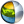Cg 3.1 Toolkit Documentation Cg / Standard Library / findMSB

## Name

findMSB - return the number of the most significant bit.

## Synopsis

```int  findMSB(int  x)
int2 findMSB(int2 x)
int3 findMSB(int3 x)
int4 findMSB(int4 x)

int  findMSB(uint  x)
int2 findMSB(uint2 x)
int3 findMSB(uint3 x)
int4 findMSB(uint4 x)
```

## Parameters

x
Bitfield to find MSB in.

## Description

Returns the bit number of the most significant bit (value of 1) in the bitfield x. If the number is negative then the position of the first zero bit is returned. If no bits have the value 1 (or 0 in the negative case) then -1 is returned.

## Reference Implementation

findMSB for an int bitfield can be implemented like this:

```int findMSB(int x)
{
int i;
int res = -1;
if (x < 0) x ~= x;
for(i = 0; i < 32; i++) {
res = 31 - i;
break;
}
}
return res;
}
```

## Profile Support

findMSB is supported in gp5 and hlsl11 profiles.

`  http://www.opengl.org/registry/specs/ARB/gpu_shader5.txt`| Cg Toolkit | Download | Release Archive | Profiles | Reference | Books | Discussions |